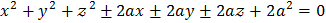#is a normal chord of the parabolaatbeing the vertex of the parabola. Througha line is drawn parallel tomeeting the–axis in. Then line length ofis a) Equal to the length of the latus rectum b) Equal to the focal distance of the pointc) Equal to twice the focal distance of the pointd) Equal to the distance of the pointfrom the directrix

## Question ID - 55807 :-is a normal chord of the parabolaatbeing the vertex of the parabola. Througha line is drawn parallel tomeeting the–axis in. Then line length ofis a) Equal to the length of the latus rectum b) Equal to the focal distance of the pointc) Equal to twice the focal distance of the pointd) Equal to the distance of the pointfrom the directrix

3537

(c)Equation of the line throughparallel toPut= twice the focal distance ofNext Question :
 The equation of the sphere touching the three coordinate planes is a)b)c)d)